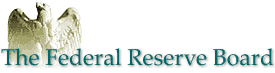Should We Expect Significant Out-of-Sample Results When Predicting Stock Returns

NOTE: International Finance Discussion Papers are preliminary materials circulated to stimulate discussion and critical comment. References to International Finance Discussion Papers (other than an acknowledgment that the writer has had access to unpublished material) should be cleared with the author or authors. Recent IFDPs are available on the Web at http://www.federalreserve.gov/pubs/ifdp/. This paper can be downloaded without charge from the Social Science Research Network electronic library at http://www.ssrn.com/.

Abstract:

Using Monte Carlo simulations, I show that typical out-of-sample forecast exercises for stock returns are unlikely to produce any evidence of predictability, even when there is in fact predictability and the correct model is estimated.

JEL classification: C15; C53; G14.

Keywords: Stock return predictability; Out-of-sample tests.

1  Introduction

Forecasting models play a central role in economics and finance, both for practitioners and academics. Although standard, in-sample, econometric methods are usually relied upon to judge the validity of a given model, out-of-sample tests are often deemed to provide the most robust assessment of any econometric forecasting model. Goyal and Welch (2003,2004) provide perhaps the most noted expression of this belief in recent times; they argue that virtually all variables that have been proposed as predictors of future stock returns provide no predictive gains in out-of-sample exercises.

Should one interpret these findings by Goyal and Welch as conclusive evidence that stock returns are not predictable? There are several reasons to adopt a more nuanced view. Inoue and Kilian (2004) show that out-of-sample tests typically have lower power than in-sample tests. They also argue that the widely held belief that out-of-sample tests are less susceptible to data-mining is not generally true. Campbell and Thompson (2005) take a different approach and show that by imposing some common sense restrictions when forming the out-of-sample forecasts, there is in fact fairly strong evidence of out-of-sample predictive ability in stock returns.

This note adds to the above strand of literature by reporting the results from simulated out-of-sample exercises. I show that the results of Goyal and Welch (2003,2004) do not imply that previous in-sample results are spurious. In fact, using Monte Carlo simulations, similar out-of-sample results to those of Goyal and Welch are found even when the postulated forecasting model is in fact the true data generating process. This result stems from the fact that any predictive component in stock returns must be small, if it does exist. Therefore, if the predictive relationship is estimated poorly, the conditional forecasting model will be outperformed by the unconditional benchmark model which assumes that expected returns are constant over time. Put in other words, in order to produce good forecasts when the slope coefficient in a linear regression is small, you are often better off setting it equal to zero, rather than using a noisy estimate of it. To accurately estimate a very small coefficient, large amounts of data are needed. The results in this paper show that when testing for stock return predictability, the sample sizes in most relevant cases are simply too small relative to the size of the slope coefficient for any predictive ability to show up in out-of-sample exercises.

The findings in this paper, of course, do not tell us that stock returns are predictable, but merely that we should not disregard the econometric in-sample results in favour of out-of-sample results. As shown here, when the level of predictability is low, it is quite possible to correctly specify and estimate the true predictive relationship but without obtaining any improvement in out-of-sample forecasts. However, this raises the question of the practical use of identifying a predictive relationship if, in fact, that relationship cannot be used to improve upon forecasts. It is evident that much care must be used in forming the forecast if it is to perform better than a simple unconditional alternative. For instance, Campbell and Thompson (2005), manage to improve the out-of-sample performance substantially by imposing some simple economically motivated restrictions on the forecasts. On the econometric side, it is of course also important to form the best possible estimate of the relevant parameters in the model. At present, most econometric studies on stock return predictability have focused primarily on the issues of testing, rather than point estimation. Hopefully, there will be also be great advances in the estimation area over the next few years.

2  Simulation design

To create simulated samples of stock-returns and predictor variables, I rely on the standard data generating process (dgp) most often found in the stock return predictability literature (e.g. Campbell and Yogo, 2005). Let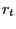denote the excess stock return in period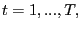and let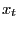denote the corresponding value of some scalar predictor variable, such as the dividend- or earnings-price ratio. The variablesandare generated according to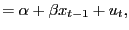(1)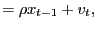(2)

where the joint innovations,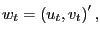are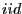normal with unit variance and correlation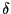. The autoregressive root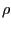is modelled as local-to-unity and takes values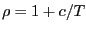, where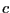is the so-called local-to-unity parameter.

To capture the often large negative correlation between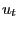and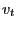, the parameteris set equal to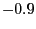. The local-to-unity-parameter is set equal to either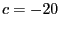or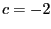and the sample size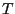is set equal to either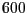or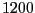to represent sample sizes of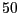or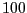years of monthly data.2 The intercept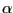is set equal to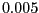and I let the slope-coefficient vary between zero and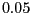. Campbell and Yogo (2005), who analyze predictability in aggregate U.S. stock returns, present their empirical results in a format standardized to conform with a unit variance in the innovationsandand show that in most cases the OLS estimate of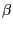is between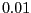and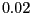for monthly data.

The aim of the Monte Carlo study is to compare the out-of-sample forecasts ofbased on an estimate of equation (1) to those based on a model of constant expected returns (i.e.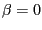). These forecasts will be referred to as the conditional and unconditional forecasts, respectively.

The simulated out-of-sample exercises are performed in the following manner. The first half of the sample is used to form the initial estimates of the conditional and unconditional models. The estimate of the unconditional model is, of course, merely the mean of the returns observed up to that point in time. For each time-period in the remaining half of the sample, the one-step-ahead forecasts based on the conditional and unconditional models are calculated and the estimates of the forecast models are updated using the additional data that become available to the forecaster every period. The slope coefficients are estimated using standard OLS. The mean squared errors (MSE) from every forecast are calculated, as well as the corresponding Diebold and Mariano (1995) (DM) statistic, which tests the null hypothesis of no additional predictive accuracy in the conditional forecast compared to the unconditional one. In order to assess the impact of poorly estimated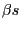, I also form conditional forecasts using the true value of. The value ofis still estimated, however. All simulation results are based on 10,000 repetitions.

3  Results

The results of the paper are presented in Figures 1 and 2, which show the Monte Carlo evidence from samples with sizes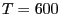and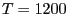, respectively. The top two panels in both figures give the results corresponding to, and the two lower panels correspond to.

Panel (a) in Figure 1 shows the ratios between the MSEs for the unconditional and conditional forecasts, when. When, on average, the conditional forecast outperforms the unconditional one, this ratio is greater than one, and vice versa. As is evident from the plot, when the conditional forecast is based on the OLS estimate of, the true value ofneeds to be greater than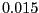for the conditional forecast to outperform the unconditional one on average. The conditional forecast based on the true value ofdoes, of course, always outperform the unconditional one. The same results forare shown in Panel (c). The conditional forecast, based on the OLS estimates, now perform better relative to the unconditional one; a true value ofgreater than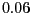is sufficient for the conditional forecast to beat the unconditional one on average. Panels (b) and (d) show the rejection rates for a one-sided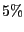DM test of higher accuracy in the conditional forecast versus the unconditional forecast, based on the MSE.3 Clearly, when using OLS estimates ofto form the conditional forecasts, the DM test lacks power to reject the null hypothesis of equal forecasting ability in the relevant regions of the parameter space. For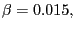the rejection rates are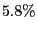and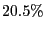forand, respectively. The chance of detecting predictive ability through the DM test is thus extremely limited whenis small. Indeed, even when the true value ofis used in forming the conditional forecasts the rejection rates remain low.

In Figure 2, the Monte Carlo results from samples with sizes, representing 100 years of monthly data, are reported. As expected, the conditional forecasts based on OLS estimates ofperform substantially better than in thecase. A value ofgreater than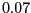, for, and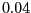for, is sufficient for the conditional forecast to outperform the unconditional one, on average. For, the DM test statistic is still not very powerful, although forthere is now a fair chance of rejecting the null for reasonable parameter values; for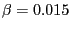the rejection rates are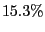and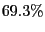, forand, respectively.

To sum up, in a monthly sample spanning 50 years it is often difficult to detect any predictive ability, whenis small, even under such perfect circumstances as in a controlled Monte Carlo experiment where the true functional form of the model is known and completely stable over time. In reality, the model is likely to be only, at best, a decent approximation of the true data generating process, which is also not likely to remain unchanged over a 50 year time span. This point, of course, is even more valid for the 100 year sample, where a stable model for the entire time span seems even less probable. Practical limitations on data availability also often restrict researchers to time spans of around 50 years or shorter. For instance, use of the short interest rate as a predictor is typically only considered for data after 1952 when the Fed unpegged the interest rate, and accounting variables, such as the book-to-market ratio, are often only available under an even shorter period.

The overall interpretation of these results must be that, in practice, it should be difficult to detect any out-of-sample predictability in stock returns, even without any demands for statistical significance but merely as evaluated by the MSE.

References

1. Andrews, D.W.K., and C.J. Monahan, 1992. An Improved Heteroskedasticity and Autocorrelation Consistent Covariance Matrix Estimator, Econometrica 60, 953-966.

2. Campbell., J.Y., and S.B. Thompson, 2005. Predicting the Equity Premium Out of Sample: Can Anything Beat the Historical Average?, Working Paper, Harvard University.

3. Campbell, J.Y., and M. Yogo, 2005. Efficient Tests of Stock Return Predictability, forthcoming Journal of Financial Economics.

4. Diebold, F.X., and R.S., Mariano, 1995. Comparing predictive accuracy, Journal of Business and Economics Statistics 13, 253-263.

5. Goyal, A., and I. Welch, 2003. Predicting the equity premium with dividend ratios, Management Science 49, 639-654.

6. Goyal, A., and I. Welch, 2004. A comprehensive look at the empirical performance of equity premium prediction, NBER Working Paper 10483.

7. Inoue, A., and L. Kilian, 2004. In-sample or out-of-sample tests of predictability: which one should we use?, forthcoming Econometric Reviews.

Figure 1

Results from Monte Carlo simulations with a simulated monthly sample of 600 observations. The top two panels show the results forand the bottom panels for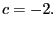The left panels, (a) and (c), display the ratios between the mean squared errors (MSE) for the unconditional and conditional forecasts; a ratio greater than one implies that the conditional forecast outperforms the unconditional one. The right hand panels show therejection rates for the Diebold and Mariano (DM) test of higher accuracy in the conditional forecast versus the unconditional forecast, based on the MSE. The dashed lines show the results for conditional forecasts based on the OLS estimate ofand the dotted lines the results for conditional forecasts based on the true value of. The flat lines in the left hand graphs indicate a value of one.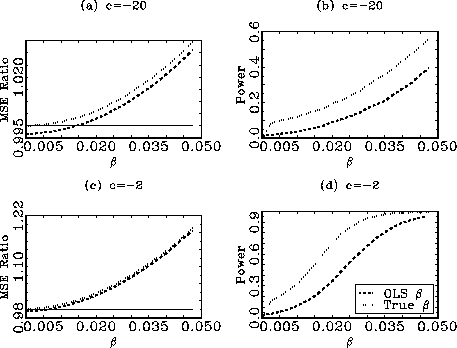Figure 2

Results from Monte Carlo simulations with a simulated monthly sample of 1200 observations. The top two panels show the results forand the bottom panels forThe left panels, (a) and (c), display the ratios between the mean squared errors (MSE) for the unconditional and conditional forecasts; a ratio greater than one implies that the conditional forecast outperforms the unconditional one. The right hand panels show therejection rates for the Diebold and Mariano (DM) test of higher accuracy in the conditional forecast versus the unconditional forecast, based on the MSE. The dashed lines show the results for conditional forecasts based on the OLS estimate ofand the dotted lines the results for conditional forecasts based on the true value of. The flat lines in the left hand graphs indicate a value of one.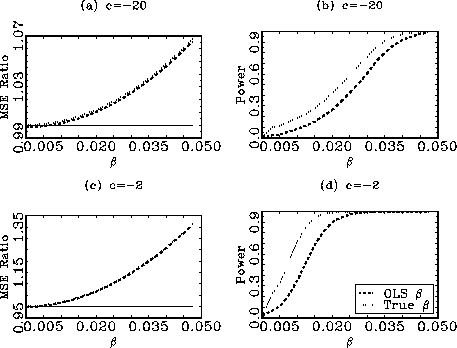Footnotes

1.  Tel.: +1-202-452-2436; fax: +1-202-263-4850; email: erik.hjalmarsson@frb.gov. The views presented in this paper are solely those of the author and do not represent those of the Federal Reserve Board or its staff. Return to text

2.  Simulations based on annual parameter values, using sample sizes ofandyears were also performed, but not reported here. These simulations delivered qualitatively identical results to the monthly ones presented here. Return to text

3.  The DM statistic is calculated using the long-run variance estimator of Andrews and Monahan (1992) with a quadratic spectral kernel. Return to text